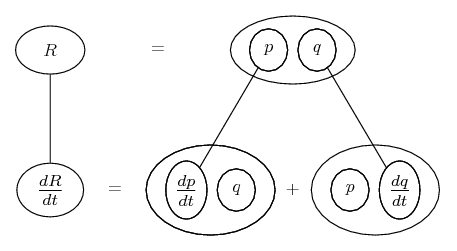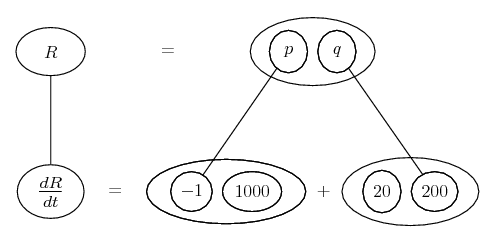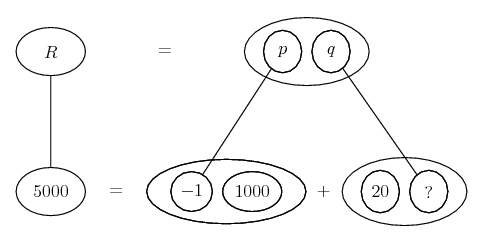# Thread: At what rate is revenue rising or falling.

1. ## At what rate is revenue rising or falling.

Problem:

"For the past few months, OHaganBooks.com has seen its weekly sales increase. At the moment OHaganBooks.com is selling 1000 books per week and its sales are rising at a rate of 200 books per week. Also, it is now selling all its book for $20 each, but the price is dropping at a rate of$1 per week. I need to know at what rate OHaganBooks.com's revenue is rising or falling given these conditions. I would also like to see that company's revenue increase at a rate of $5,000 per week. At what rate would sales have to have been increasing to accomplish this?" So those are the two questions I have to answer. Pretty simple I'm guessing. I understand (R=pq) which is not hard to calculate for each week. I put the answers out in a table showing the difference in revenue from the previous week, and the total revenue for the week. However, I'm guessing there is some calculus technique I need to use here and I'm not quite sure what it is. If anyone could take a look at it and see what they think I would greatly appreciate it. 2. The rates to relate are of R, p and q, and since R=pq we can see that differentiating them all with respect to t will involve the product rule. I would think...... where... is the product rule - straight continuous lines differentiating downwards (integrating up) with respect to t. Spoiler:Spoiler:__________________________________________ Don't integrate - balloontegrate! Balloon Calculus: standard integrals, derivatives and methods Balloon Calculus Drawing with LaTeX and Asymptote! 3. Here is what I think, Let P(x) = the profit, they are selling there books 20$ a piece.
Let f(x) = the number of books they are selling per week.

f(x)=1000+200x where x is the week number.

But they sell books 20$but with price dropping of 1$ per week per book.

P(x)=1000(20)-1000*x+200(20)*x-200*x

I need to know at what rate OHaganBooks.com's revenue is rising or falling given these conditions:

Take the derivative of P(x)

P'(x)=-1000+4000-200=2800$Hence revenue are rising at a rate of 2800$ per week.

At what rate would sales have to have been increasing to accomplish this?"

You want the derivative of our function = 5 000$per week. 1000 books per week sold is fix...let u denote the rising sales. Then under the same condition: P(x)=1000*20-1000*x+u*20*x-u*x P'(x)=-1000+20*u-u=5 000 Solve for u you get: 315.79 books, hence sales have to go up of 316 books per week. 4. I appreciate the above answers, but I'm still not understanding the equations here I guess. 5. Ok, well this company is selling 1000 books a week. Moreover, the sales are raising by 200 books per week. That is, f(x)=number of book sold in one week. f(x)=1000+200x where x is the week number. They are selling the books 20$ each and the price of them is deacreasing by 1$dollar a week. That is, f(x)=1000*(20$)-1000*x+200*x*(20)-200*x where x is the week number.

In this problem, the price dropping is = to the week number...

#### Search Tags

falling, rate, revenue, rising